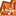# App Development

Announcements
Join us for a live Q&A! September 21, 10 AM ET - Onboarding Fast in Qlik Sense SaaS! REGISTER
cancel
Showing results for
Did you mean:Creator II

## Count # employees earning less/more than the average (per month, quarter, year)

Dear Qlik Community,

I am currently looking to count the number of employees earning less/more than the average in a given time-period (in a bar graph).

For average salary per month I have the following equation:

avg(aggr(sum({<Amount={'=sum(Amount) >1'}>}Amount), key, MonthYear))

To obtain the average per quarter or year I just replace 'MonthYear' with quarter or year.

I tried counting the # of employees earning less/ more with if statements and set analysis:

Attempt 1:

count ( {< key = {" avg(aggr(sum({<Amount={'=sum(Amount) >1'}>}Amount), key, MonthYear)) > avg(aggr(sum({<Amount={'=sum(Amount) >1'}>}Amount), key, MonthYear))"} >} distinct key)

Attempt 2:

if(avg(aggr(sum({<Amount={'=sum(Amount) >1'}>}Amount), key, MonthYear)) < avg(aggr(sum({<Amount={'=sum(Amount) >1'}>}Amount), key, MonthYear)), count (distinct key))

I realise I am doing something very wrong (beyond the syntax I can't figure out how to differentiate the average salary per month to the average salary of the specific person so that teh <  or >  exclusions works)  ! I can't seem to figure this out. Any advice would be very much appreciated 🙂

Best,

Mohammed

Labels (4)

• ### Set Analysis

1 Solution

Accepted SolutionsMVP

Are you trying this may be?

``````Count(DISTINCT Aggr(

If(Sum({<Amount = {">1"}>} Amount) > Avg(TOTAL <MonthYear> Aggr(Sum({<Amount = {">1"}>} Amount), key, MonthYear)), key)

, key, MonthYear))``````
2 RepliesMVP

Are you trying this may be?

``````Count(DISTINCT Aggr(

If(Sum({<Amount = {">1"}>} Amount) > Avg(TOTAL <MonthYear> Aggr(Sum({<Amount = {">1"}>} Amount), key, MonthYear)), key)

, key, MonthYear))``````Creator II
Author

Thank you very much Sunny! Works perfectly.Tags
Community Browser